# Step 4 - Permutate the last layer - PLL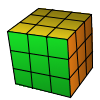The 4th and final step of the advanced Fridrich method is the permutation of the last layer (PLL). At this point the white cross, the first two layers (F2L) are both done and the last layers pieces are oriented (OLL). When we execute this last step our Rubik's Cube will be solved.

Again, X and Y (x,y) are whole cube rotations, while lowercase u is double layer turn.

First rotate the top layer to align as many pieces as possible then do one of the 21 algorithms listed below. If this seems too many to learn, you should try the 2look PLL which method contains only six algorithms but takes more time to execute.

Here are four animated examples and find the complete list below.

A 1
U 1
H
T

I wrote the name of each algorithm at the beginning of the rotation chain. On the images the dots mark the direction of the permuataitons (like arrowheads).A 1: x [(R' U R') D2] [(R U' R') D2] R2A 2: x' [(R U' R) D2] [(R' U R) D2] R2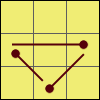U 1: R2 U [R U R' U'] (R' U') (R' U R')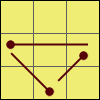U 2: [R U'] [R U] [R U] [R U'] R' U' R2H: M2 U M2 U2 M2 U M2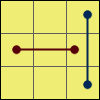T: [R U R' U'] [R' F] [R2 U' R'] U' [R U R' F']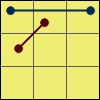J 1: [R' U L'] [U2 R U' R' U2] [R L U']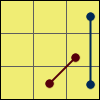J 2: [R U R' F'] {[R U R' U'] [R' F] [R2 U' R'] U'}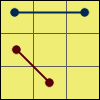R 1: [L U2' L' U2'] [L F'] [L' U' L U] [L F] L2' U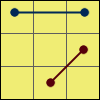R 2: [R' U2 R U2] [R' F] [R U R' U'] [R' F'] R2 U'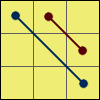V: [R' U R' d'] [R' F'] [R2 U' R' U] [R' F R F]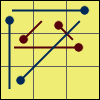G 1: R2 u R' U R' U' R u' R2 [y' R' U R]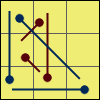G 2: [R' U' R] y R2 u R' U R U' R u' R2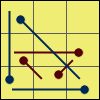G 3: R2 u' R U' R U R' u R2 [y R U' R']G 4: [R U R'] y' R2 u' R U' R' U R' u R2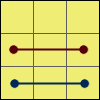F: [R' U2 R' d'] [R' F'] [R2 U' R' U] [R' F R U' F]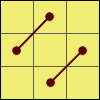Z: M2 U M2 U M' U2 M2 U2 M' U2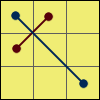Y: F R U' R' U' [R U R' F'] {[R U R' U'] [R' F R F']}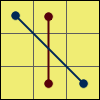N 1: {(L U' R) U2 (L' U R')} {(L U' R) U2 (L' U R')} UN 2: {(R' U L') U2 (R U' L)} {(R' U L') U2 (R U' L)} U'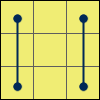E: X' (R U' R') D (R U R') u2 (R' U R) D (R' U' R)